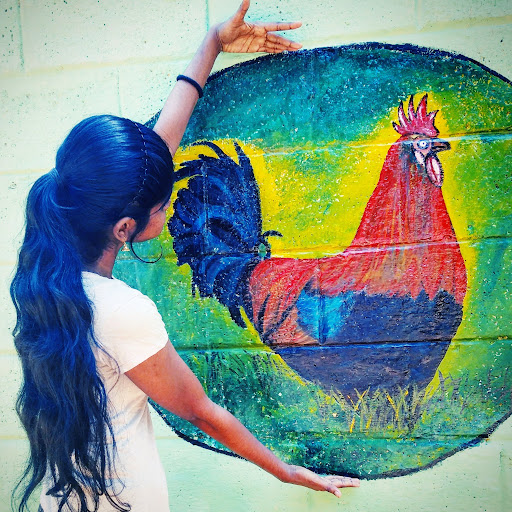# C Program for Consecutive Prime Sum (TCS Codevita) | PrepInsta## Consecutive Prime Sum Problem Solution in C

Consecutive Prime Sum is one of the challenging problem which was asked in the sample questions of TCS CodeVita 2020 Season 9 edition. TCS CodeVita is one of the toughest coding competition throughout the world. Last year there were more than 2 lakhs coders who participated in this contest, and only 25 coders reached the grand finale round. Here you will find the solution of Consecutive Prime Sum problem in C programming Language

## Problem Description

Question – :  Some prime numbers can be expressed as a sum of other consecutive prime numbers.

• For example
• 5 = 2 + 3,
• 17 = 2 + 3 + 5 + 7,
• 41 = 2 + 3 + 5 + 7 + 11 + 13.
Your task is to find out how many prime numbers which satisfy this property are present in the range 3 to N subject to a constraint that summation should always start with number 2.

Write code to find out the number of prime numbers that satisfy the above-mentioned property in a given range.

Input Format: First line contains a number N

Output Format: Print the total number of all such prime numbers which are less than or equal to N.

Constraints: 2<N<=12,000,000,000

S.no Input Output Comment
1 20 2 (Below 20 there are two such members; 5 and 17) 5=2+3 17=2+3+65+7
2 15 1

## C Code for Consecutive Prime Sum Problem

```#include  <stdio.h>
int prime(int b);
int main()
{
int i,j,n,cnt,a,c,sum=0,count=0,k=0;
printf("Enter a number : ");
scanf("%d",&n);
for(i=2;i<=n;i++)
{
cnt=1;
for(j=2;j<=n/2;j++)
{
if(i%j==0)
cnt=0;
}
if(cnt==1)
{
a[k]=i;
k++;
}
}
for(i=0;i<k;i++)
{
sum=sum+a[i];
c= prime(sum);
if(c==1)
count++;
}
printf(" %d",count);
return 0;
}

int prime(int b)
{
int j,cnt;
cnt=1;
for(j=2;j<=b/2;j++)
{
if(b%j==0)
cnt=0;
}
if(cnt==0)
return 1;
else
return 0;
}```
```Output
Enter a number : 43
4```

## Python

To find the solution of Consecutive Prime sum problem in Python Programming language click on the button below:

## C++

To find the solution of Consecutive Prime sum problem in C++ Programming language click on the button below:

C++

## Java

To find the solution of Consecutive Prime sum problem in Java Programming language click on the button below:

Java

### One comment on “C Program for Consecutive Prime Sum (TCS Codevita) | PrepInsta”

•Angel

Here count will increase for 2+3+5=10 not a prime, then how could we consider this for the count for sum of prime…can anyone plz explain it 🤯🤕??
for(i=0;i<k;i++)
{
sum=sum+a[i];
c= prime(sum);
if(c==1)
count++;
}
printf(" %d",count);
return 0;
}

int prime(int b)
{
int j,cnt;
cnt=1;
for(j=2;j<=b/2;j++)
{
if(b%j==0)
cnt=0;
}
if(cnt==0)
return 1;
else
return 0;
}1